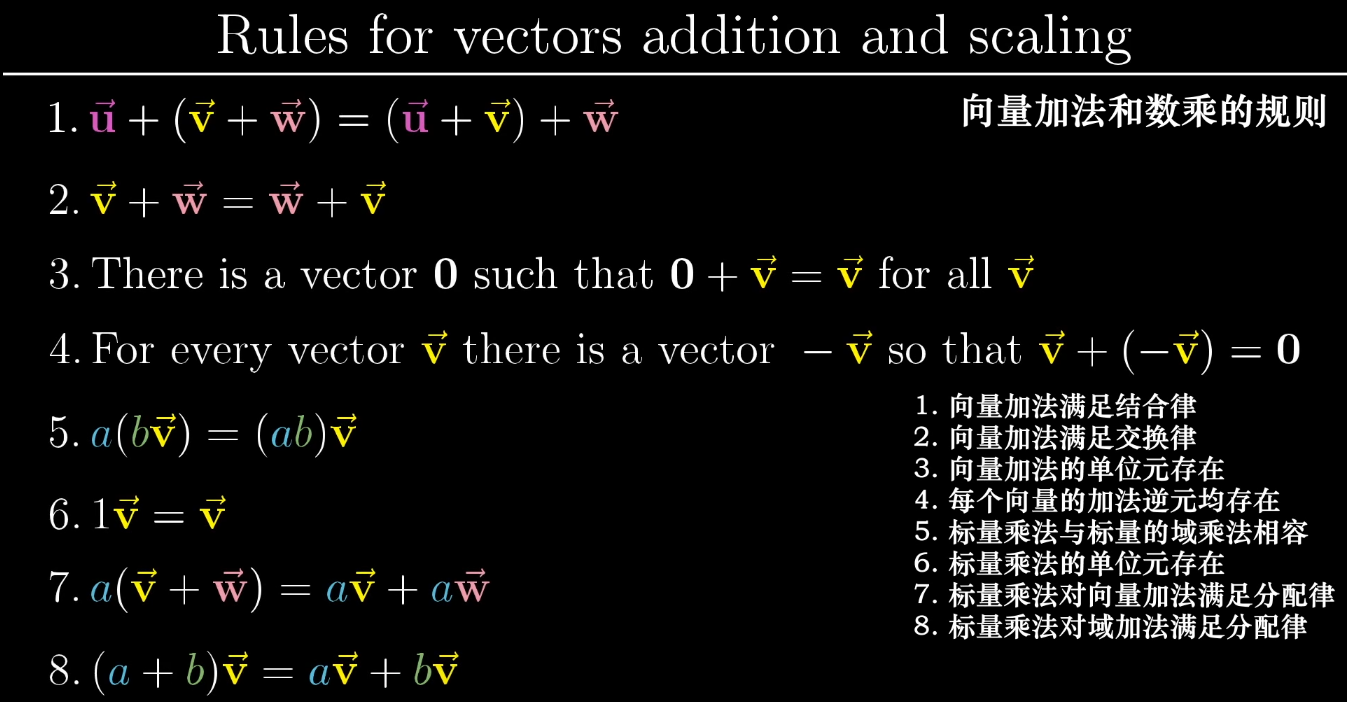【阅读时间】1小时左右 words 14069words
【内容简介】将只停留在数值运算和公式的线性代数推进到可视化几何直观（Visual Geometric Intuition）的领悟上，致敬3B1B的系列视频的笔记，动图也都来自于视频。内容涉及到基变换，叉积，逆矩阵，点积，特征向量与特征值。每一章节都有一句经典的名言，非常有启发性

$$\sin (x) = x - \frac{x^3}{3!} + \frac{x^5}{5!} + \cdots + (-1)^n\frac{x^{2n+1}}{(2n+1)!} + \cdots$$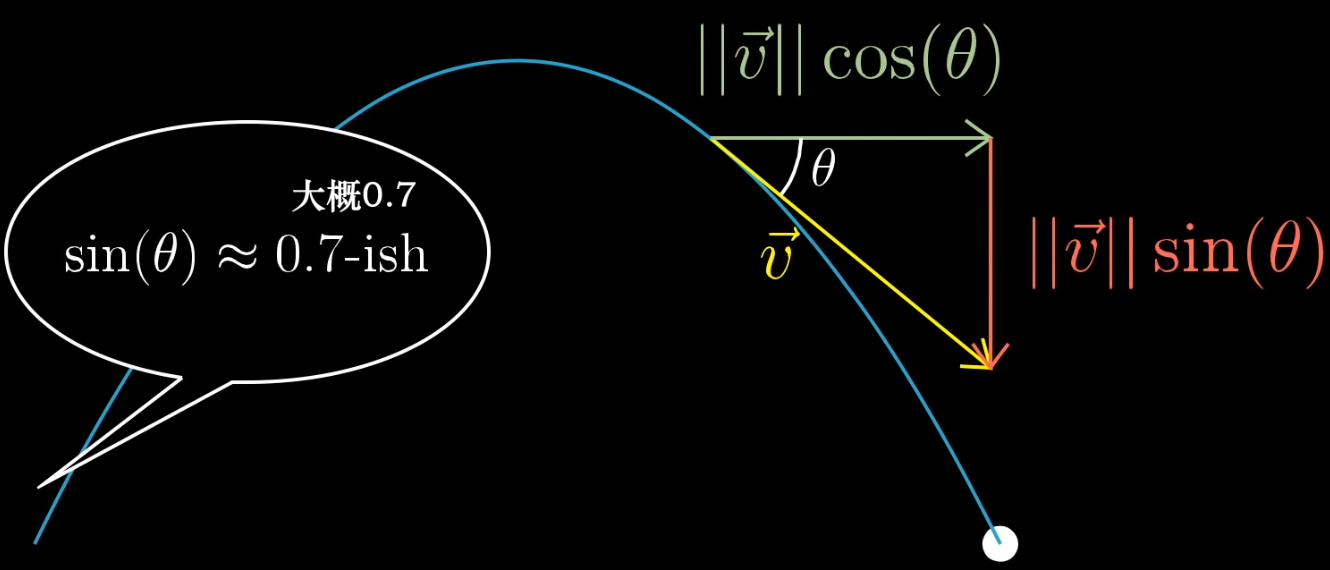# 向量究竟是什么

The introduction of numbers as coordinates is an act of violence - Hermann Weyl

## 向量的定义 What

### 物理专业角度

• 向量是空间中的箭头
• 决定一个向量的是：它的长度和它所指的方向

### 计算机专业角度

• 向量是有序的数字列表
• 向量不过是“列表”一个花哨的说法
• 向量的维度等于“列表”的长度

### 数学专业角度

• 向量可以是任何东西，只需要保证：两个向量相加及数字与向量相乘是有意义的即可
• 向量加法向量乘法贯穿线性代数始终，十分重要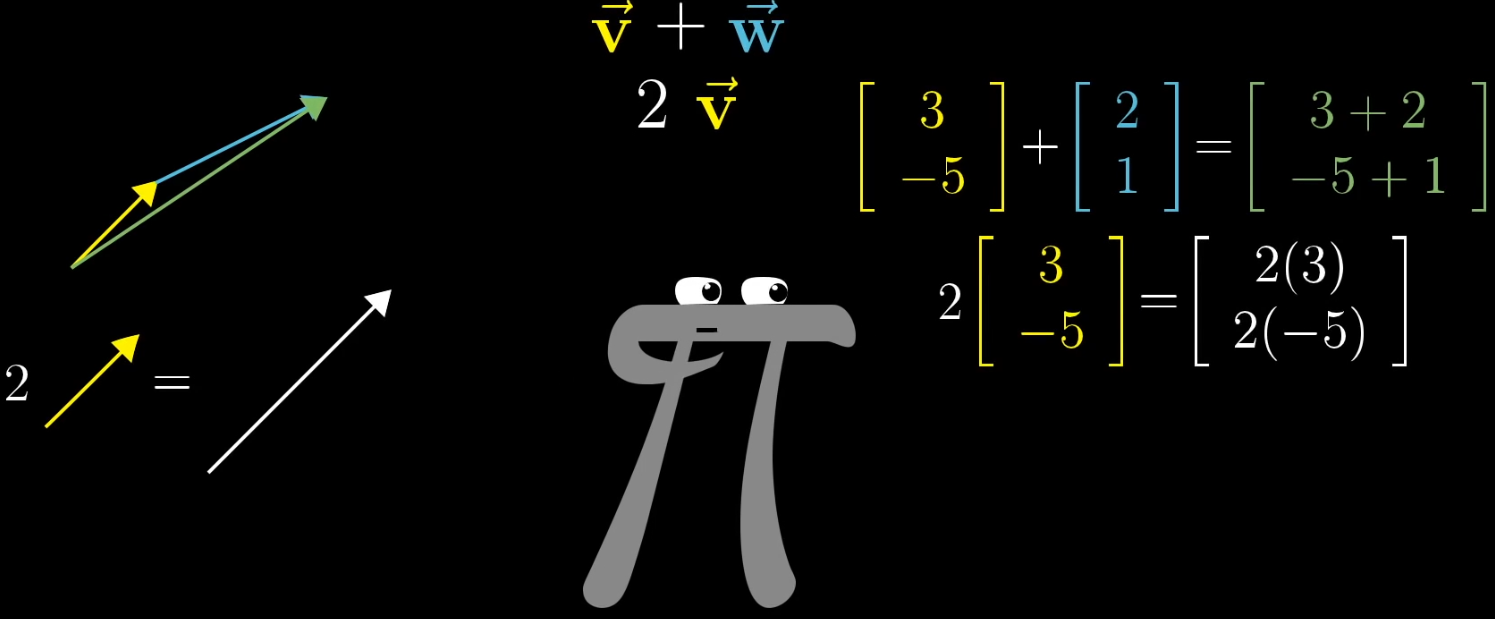## 坐标系

• 向量加法
• 物理：首尾相连 Motion
• 计算机：坐标相加
• 向量乘法
• 物理：缩放 Scaling
• 计算机：坐标和比例相乘

# 线性组合、张成的空间与基

Mathematics requires a small dose, not of genius, but of an imaginative freedom which, in a larger dose, would be insanity - Angus K. Rodgers

## 线性组合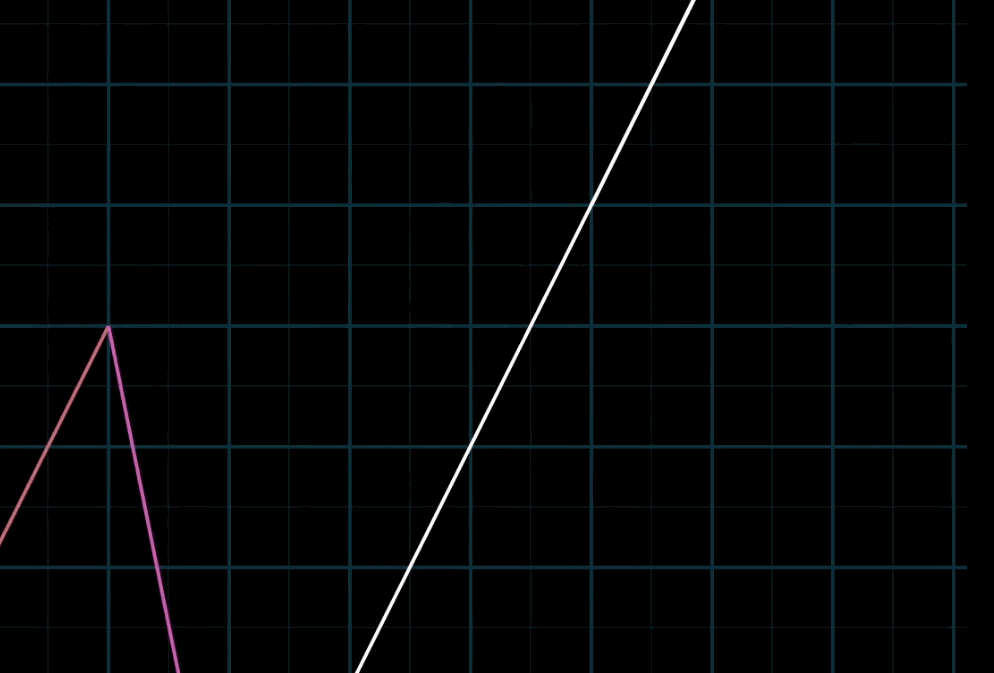## 空间的基 Basis

### 线性相关

• 【表达一】你有多个向量，并且可以移除其中一个而不减小张成的空间（即2D共线或3D共面），我们称它们（这些向量）线性相关
• 【表达二】其中一个向量，可以表示为其他向量的线性组合，因为这个向量已经落在其他向量张成的空间之中

# 矩阵与线性变换

Unfortunately, no one can be told what the Matrix is. You have to see it yourself -Morpheus

## 变换

【变换】本质上是【函数】（左）的一种花哨的说法，它接受输入内容，并输出对应结果，矩阵变换（右），同理，如下图【变换】的表达方法暗示了我们可以用运动的方法来理解【向量的函数】这一概念，可以用可视化的方法来展现这组【变换】即输入-输出关系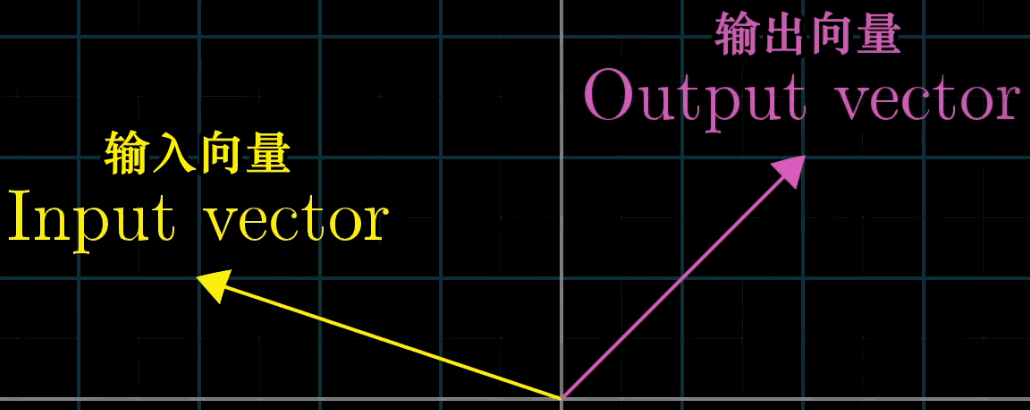## 线性变换

• 直线在变换后仍然保持为直线，不能有所弯曲
• 原点必须保持固定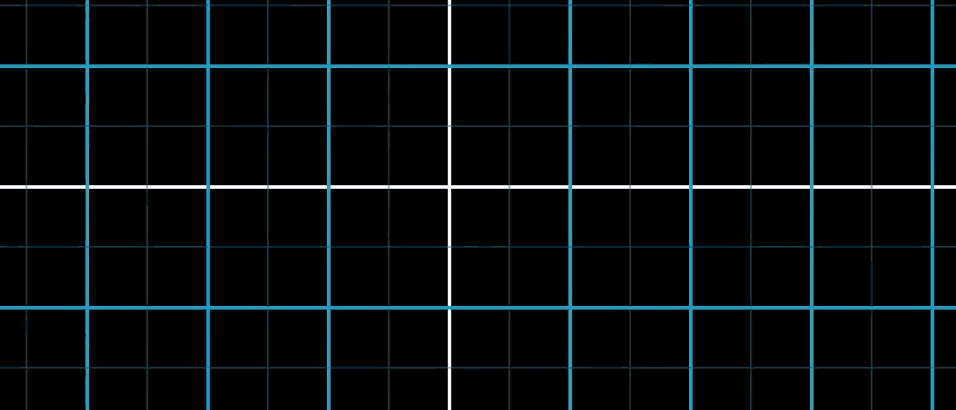## 如何用数值描述线性变换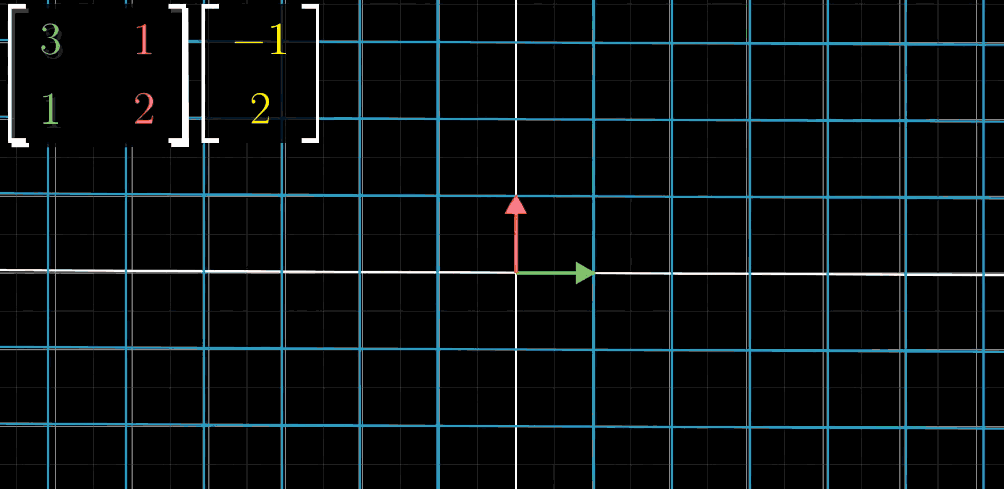Step1：绿色 $\hat {\imath}$ （x轴）进行移动（变换）
Step2：红色 $\hat {\jmath}$ （y轴）进行移动（变换）
Step3：目标向量x轴坐标值与 $\hat {\imath}$ 变换后向量进行向量乘法
Step4：目标向量y轴坐标值与 $\hat {\jmath}$ 变换后向量进行向量乘法
Step5：两者进行向量加法，得到线性变换结果

$$\begin{bmatrix} \color{green}{a}&\color{red}b \\ \color{green}c&\color{red}d \end{bmatrix} \begin{bmatrix}x\\y\end{bmatrix} = \underbrace{x \begin{bmatrix}\color{green}a\\\color{green}c \end{bmatrix} + y \begin{bmatrix} \color{red}b\\\color{red}d\end{bmatrix}}_{\text{直观的部分这里}} = \begin{bmatrix} \color{green}{a}\color{black}{x}+\color{red}{b}\color{black}{y}\\\color{green}{c}\color{black}{x}+\color{red}{d}\color{black}{y}\end{bmatrix}$$

### 【线性】的严格定义

• 【线性变换】是操纵空间的一种手段，它保持网格线平行且等距分布，并保持原点不动
• 【矩阵】是描述这种变换的一组数字，或者说一种描述线性变换的语言

$$L(\mathbf {\vec v} + \mathbf {\vec w}) = L(\mathbf{\vec v}) +L(\mathbf{\vec w}) \qquad “可加性” \\ L(c \mathbf{\vec v}) = c L(\mathbf{\vec v}) \qquad “成比例”$$

# 矩阵乘法与线性变换复合

It is my experience that proofs involving matrices can be shortened by 50% if one throws the matrices out -Emil Artin

## 复合变换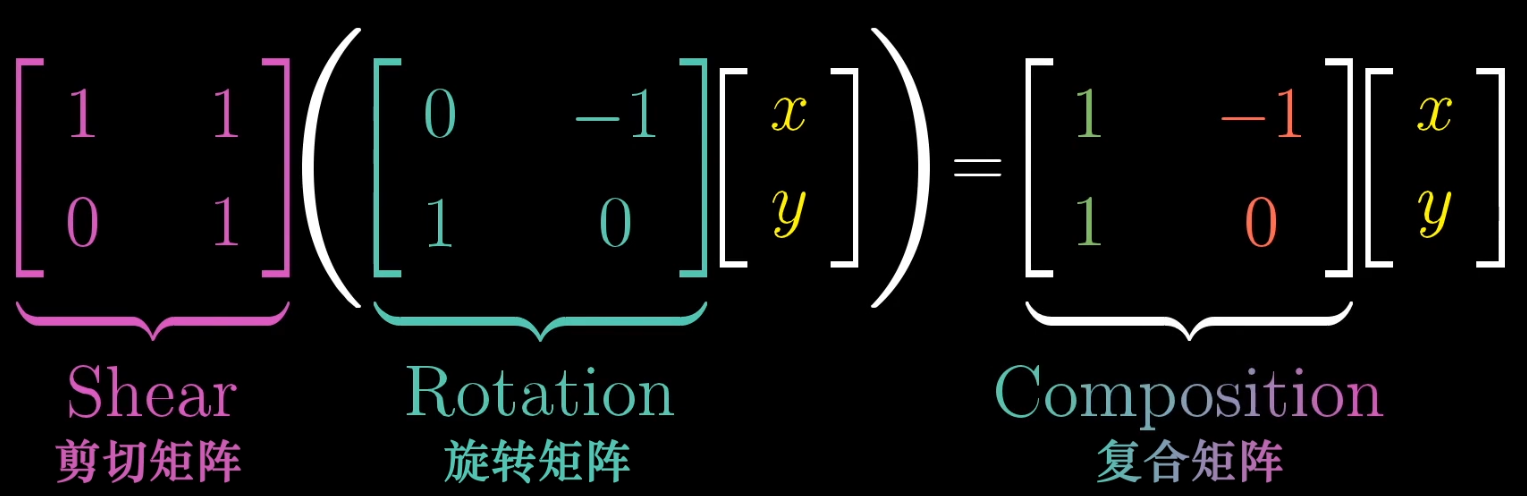## 矩阵乘法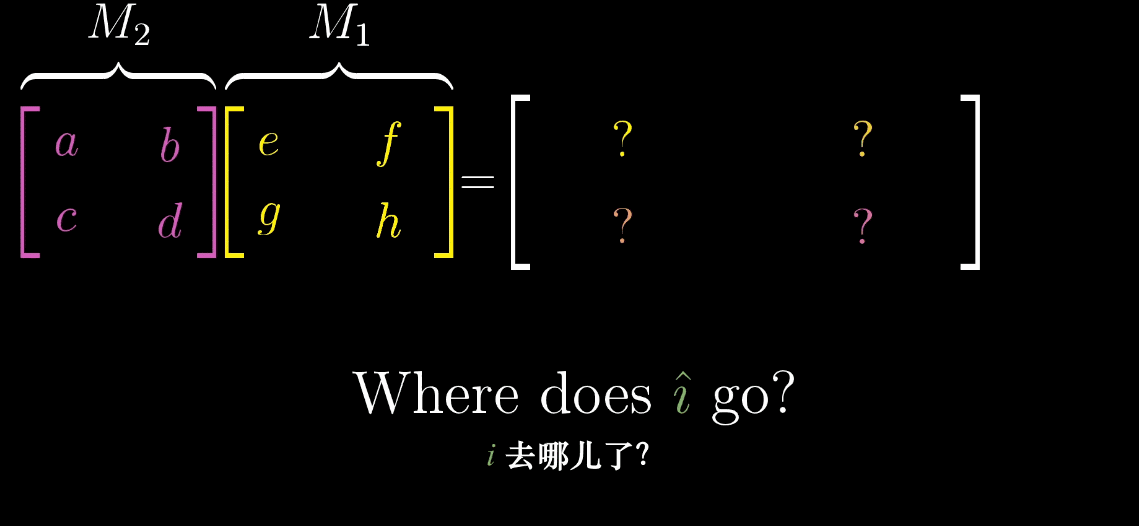M1矩阵的第一列表示的是 $\hat {\imath}$ 变换的位置，先把它拿出来，M2矩阵看成对这个变换过的 $\hat {\imath}$ 进行一次变换（按照同样的规则），就如上图所示。同理，针对 $\hat {\jmath}$ 一样的操作过程，就可以得出这个表达式。这里我也不把它写出来了，按照这种思路，并且把上面的动图多看几遍，如果还能忘记，那就要去补一补基本的对几何图形的反应能力了（这也是一种能力，包括三维想象力，心算能力，都和记忆或肌肉一样，不锻炼，是不可能躺着被提高的

# 行列式

The purpose of computation is insight, not numbers - Richard Hamming

## 行列式定义的由来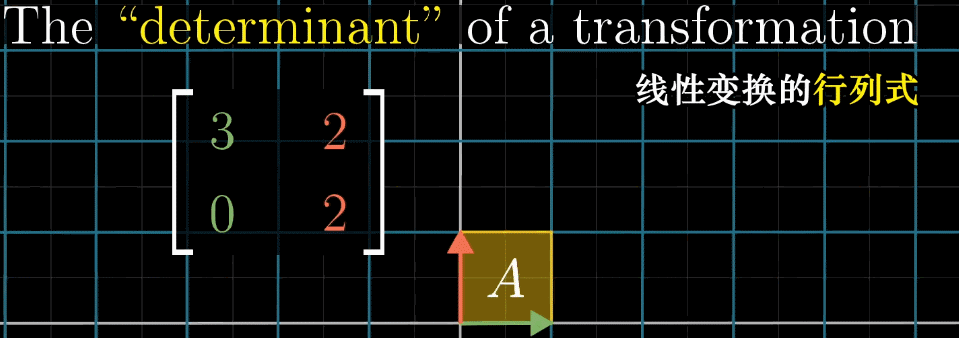## 计算行列式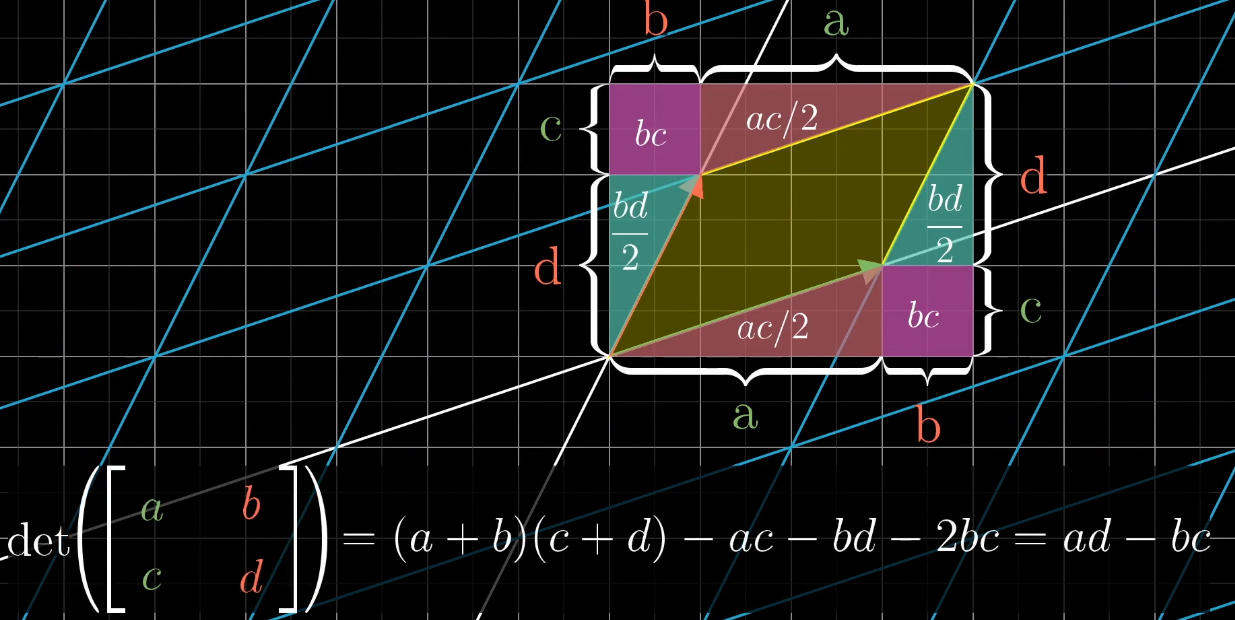# 逆矩阵、列空间与零空间

To ask the right question is harder than to answer it - Georg Cantor

## 矩阵的用途

• 描述对空间的操作，3*3矩阵描述的三维变换
• 可以帮助我们解线性方程组（Linear System of equation）

## 线性方程组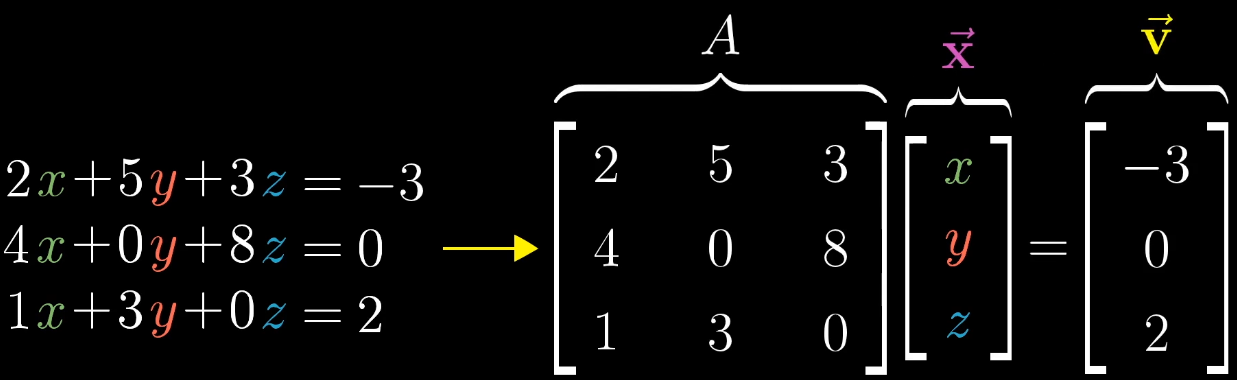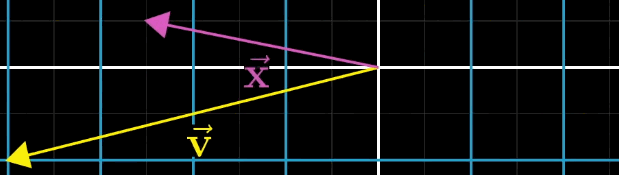$$\mathbf A^{-1}\mathbf A\mathbf{\vec x} =\mathbf A^{-1} \mathbf{\vec v} \implies \mathbf{\vec x} =\mathbf A^{-1} \mathbf{\vec v}$$

## 逆矩阵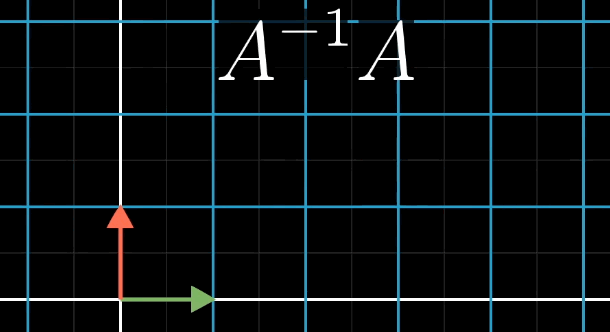## 列空间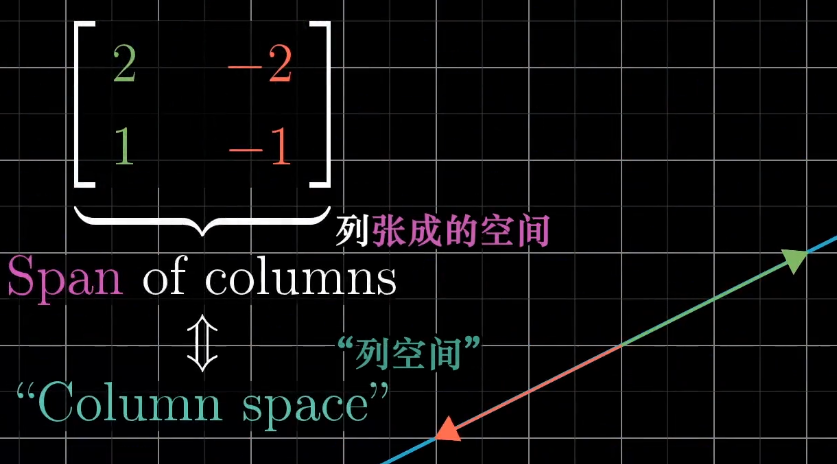## 零空间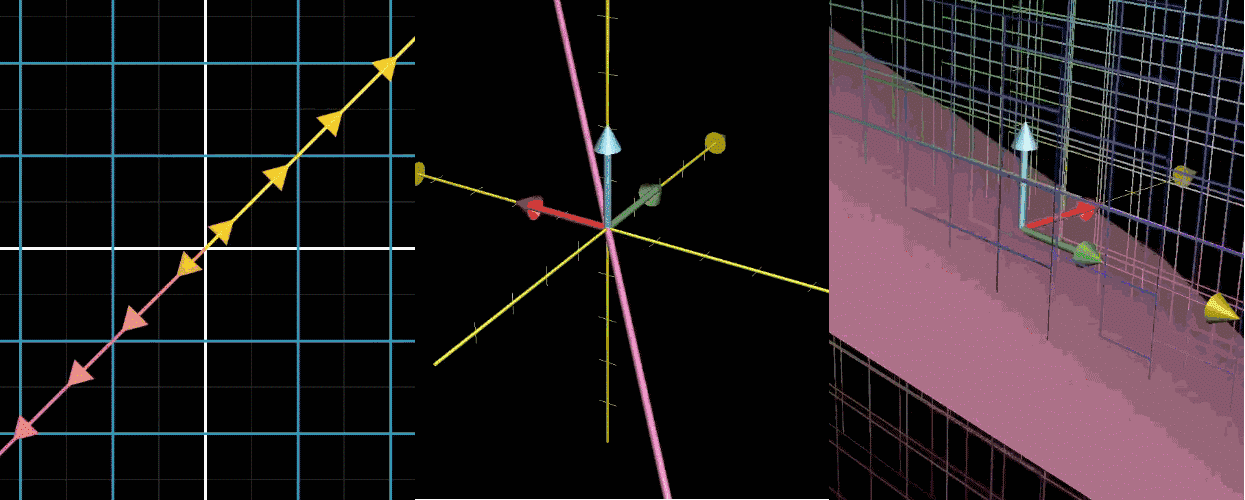【图1】二维压缩到一个直线（一维），有一条直线（一维）的点被压缩到原点
【图2】三维压缩到一个面（二维），有一条直线（一维）的点被压缩到原点
【图3】三维压缩到一条线（一维），有一条直线（二维）的点被压缩到原点

【注意】压缩就是变换，变换就是矩阵，其实说的就是矩阵

## 总结

• 几何角度理解线性方程组的一个高水平概述
• 每个方程组都有一个线性变换与之联系，当逆变换存在时，你就能用这个逆变换求解方程组
• 不存在逆变换时，列空间的概念让我们清楚什么时候存在解
• 零空间的概念有助于我们理解所有可能得解的集合是什么样的

# 非方阵

On this quiz, I asked you to find the determinant of a 2*3 matrix. Some of you, to my great amusement, actually tried to do this - no name listed

# 点积与对偶性

## 什么是点积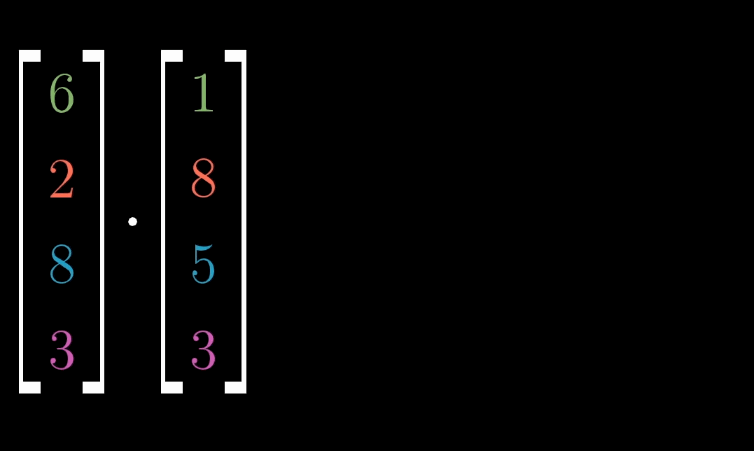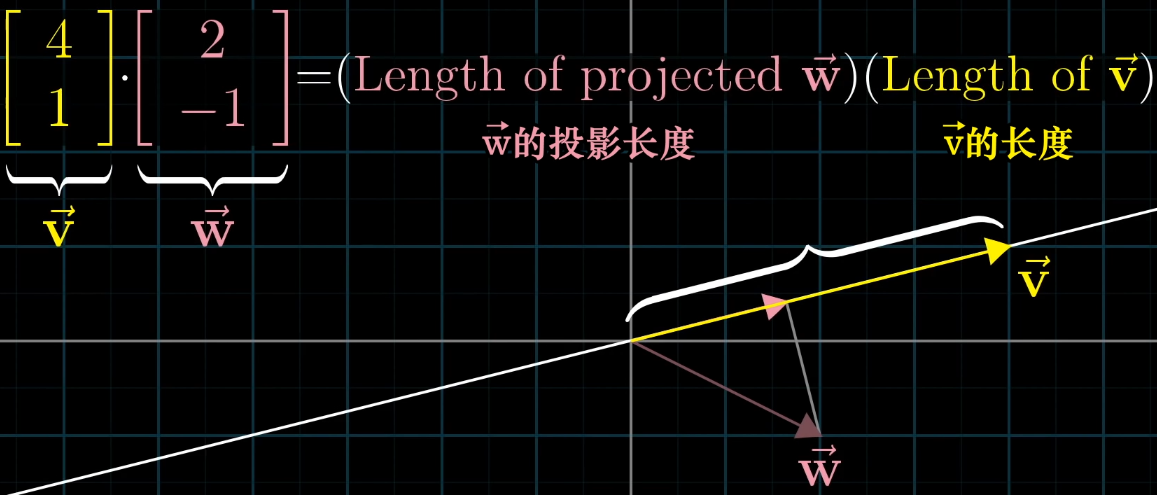## 点积的顺序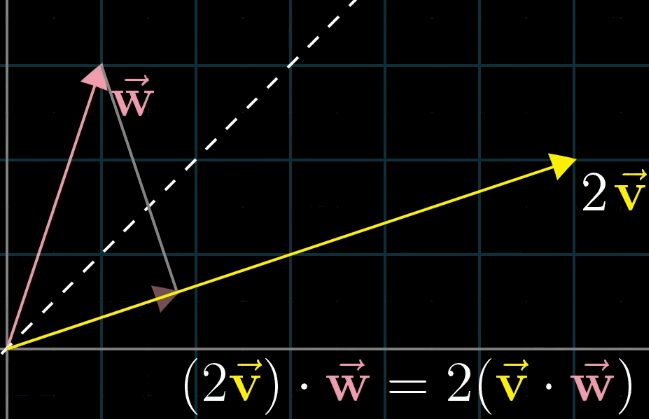## 点积与投影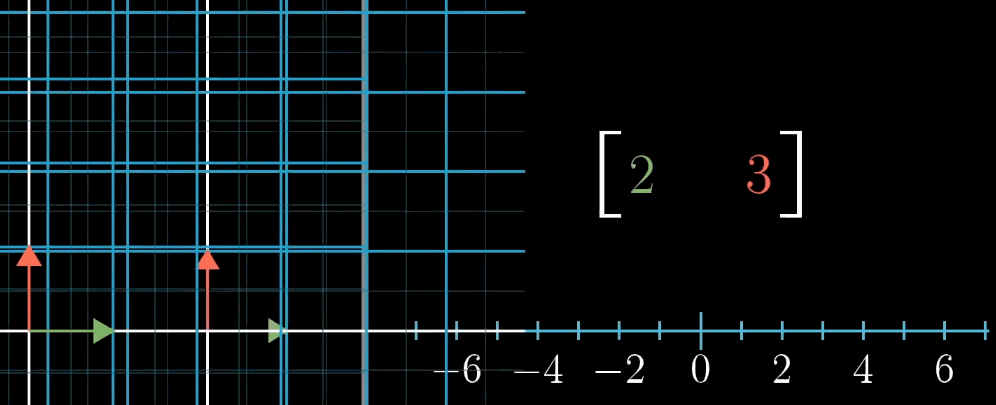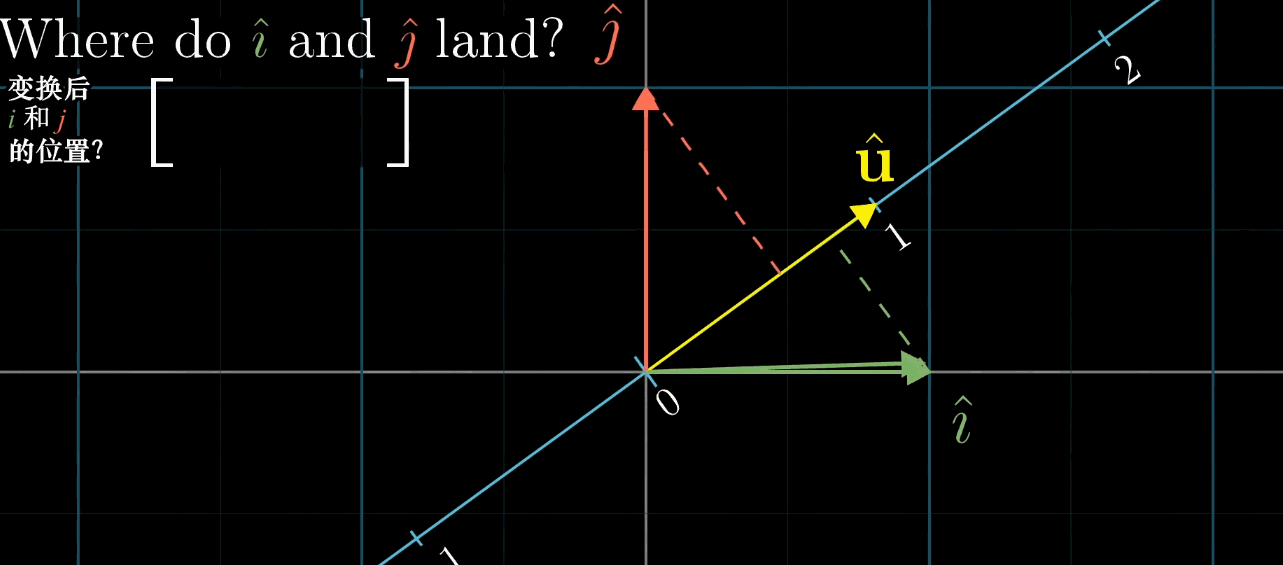## 总结

• 点积是理解投影的有力几何工具
• 方便检验两个向量的指向是否相同
• 更进一步，两个向量点乘，就是将其中一个向量转化为线性变换
• 向量放佛是一个特定变换的概念性记号。对一般人类来说，想象空间中的向量比想象这个空间移动到数轴上更加容易

# 叉积

## 二维情况下的叉积类比

$\mathbf{\vec v}$ 与 $\mathbf{\vec w}$ 张成的平行四边形的面积，即 $\mathbf{\vec v} \times \mathbf{\vec w}$ ，结果方向的确定考虑 $\hat {\imath}$ 和 $\hat {\jmath}$ 的相对位置关系，与其相同，为正；否则，为负

• 越接近垂直的 $\mathbf{\vec v}$ 与 $\mathbf{\vec w}$ 构成的面积越大
• 并且叉积的分配律成立

## 叉积计算公式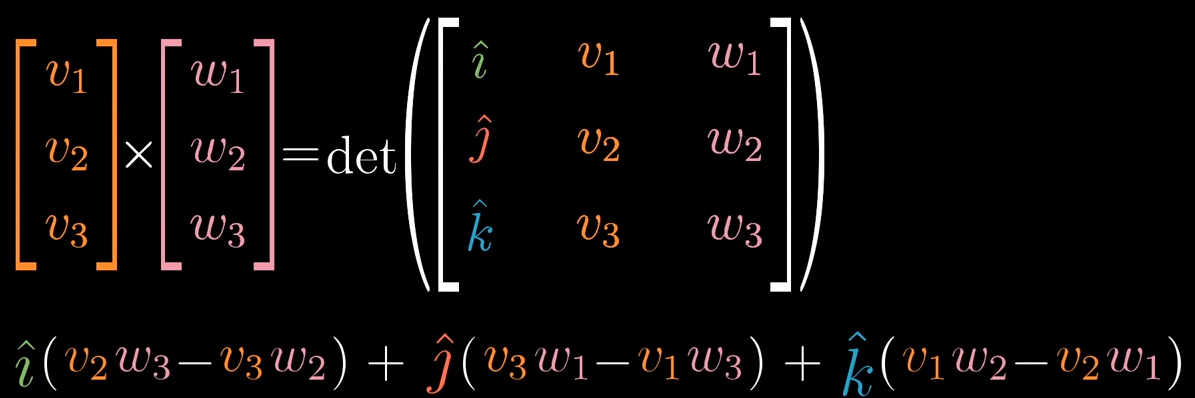## 叉积计算的几何直观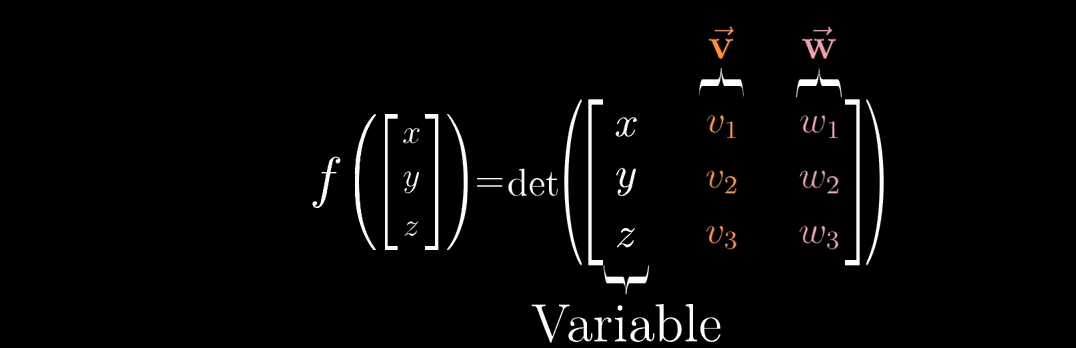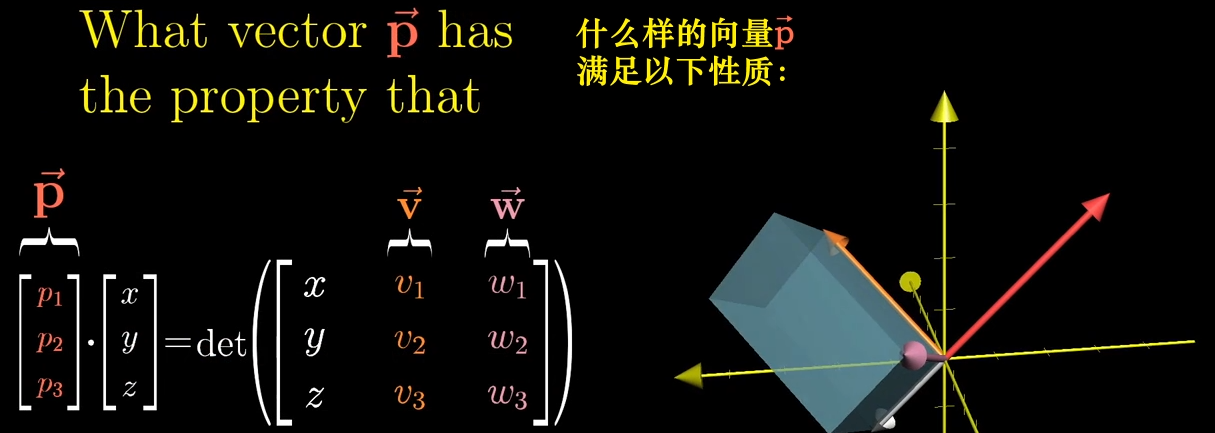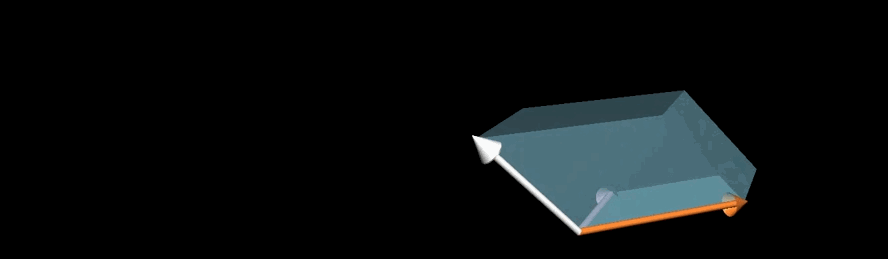## 总结

• 首先定义了一个三维空间到数轴的线性变换（函数 $f()$ ），它是根据向量 $\mathbf{\vec v}$ 和 $\mathbf{\vec w}$ 来定义的
• 接着通过两种不同的方式来考虑这个变换的对偶向量
• 这种计算方法引导你在第一列中插入 $\hat {\imath}$ $\hat {\jmath}$ $\hat k$ ，然后计算行列式
• 在几何直观上，这个对偶向量一定与 $\mathbf{\vec v}$ 和 $\mathbf{\vec w}$ 垂直，并且其长度与这两个向量张成的平行四边形的面积相同

# 基变换

Mathematics is the art of givinh the same name to different things -Henri Poincare

## 如何转化一个矩阵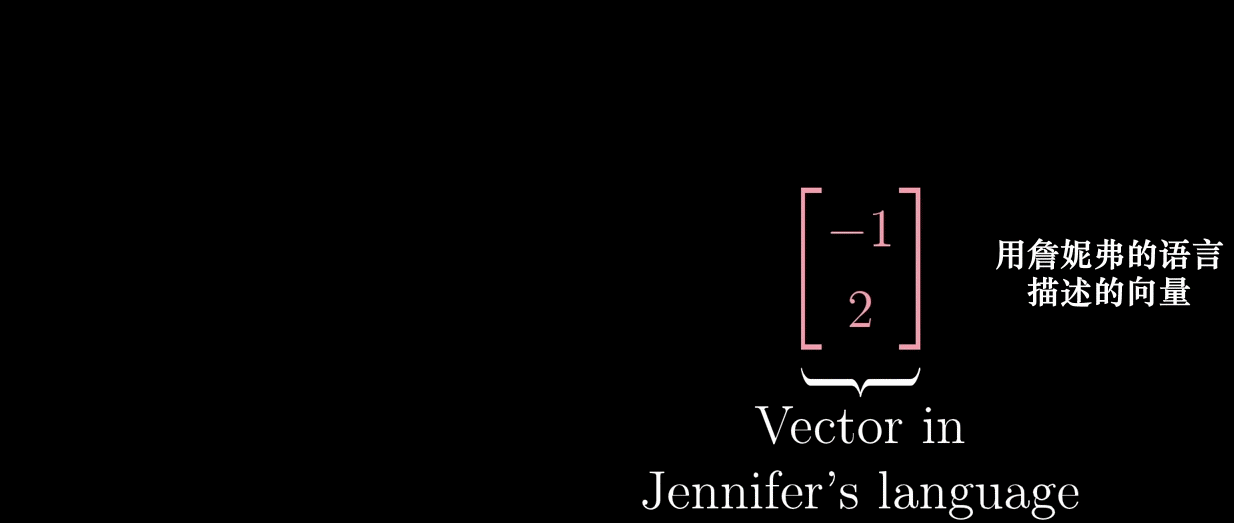• 左乘基变换矩阵（矩阵的列代表的是用我们的语言描述詹妮弗语言的基向量）：需要被转换的詹妮弗的语言：$[\begin{smallmatrix} -1 \\ 2 \end{smallmatrix}]$ ➜ 使用我们的语言描述来描述同一个向量
• 左乘线性变换矩阵（表示的变化为：左旋转90°）：➜变换的后的向量（还是以我们的语言来描述）
• 左乘基变换矩阵的逆：➜变换后的向量（用詹妮弗的语言来描述）

## 总结

• 中间的 $\mathbf M$ 代表一种你所见的转换（例子中的90°旋转变换）
• 两侧的矩阵 $\mathbf A$ 代表着转移作用（不同坐标系间的基向量转换），即就是视角上的转换
• 矩阵乘积仍然表示着同一个变换，只不过从其他人的角度来看

# 特征向量与特征值

## What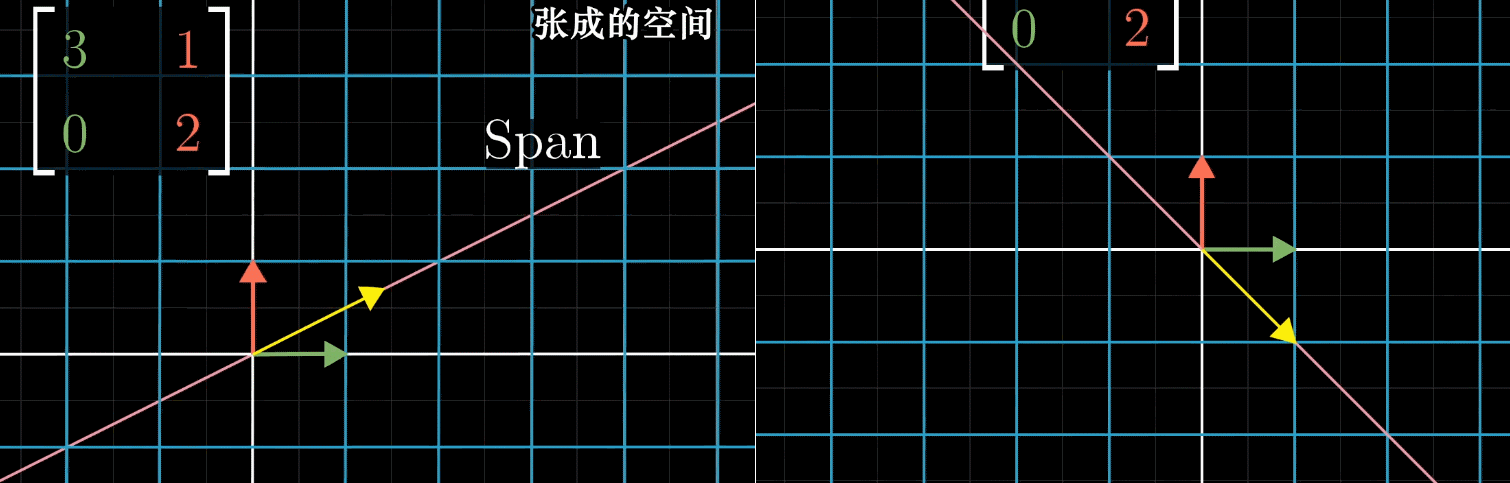• 变换中被留在张成空间内的向量，就是特征向量（上例x轴和$(-1,1)$）
• 其中每个向量被拉伸或抽缩的比例因子，就是特征值（上例3和2
• 正负表示变换的过程中是否切翻转了方向

## How

$$\mathbf A \mathbf{\vec v} = \lambda \mathbf{\vec v}$$

$\mathbf A$ 是求特征值和特征向量的变换矩阵；$\mathbf{\vec v}$ 是特征向量；$\lambda$ 是特征值；目标是找 $\mathbf{\vec v}$ 和 $\lambda$

$$\mathbf A \mathbf{\vec v} = (\lambda \mathbf I ) \mathbf{\vec v} \implies (\mathbf A - \lambda \mathbf I ) \mathbf{\vec v} = 0$$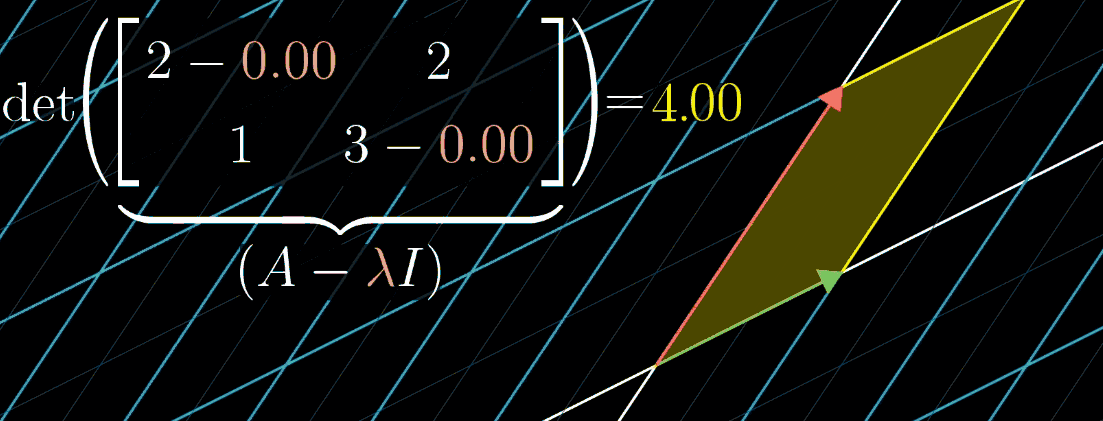## 特征向量的特殊情况

### 剪切变换

Shear变换。x轴不变，只有一个特征值，为1（$(\lambda-1)^2=0$）

## 特征基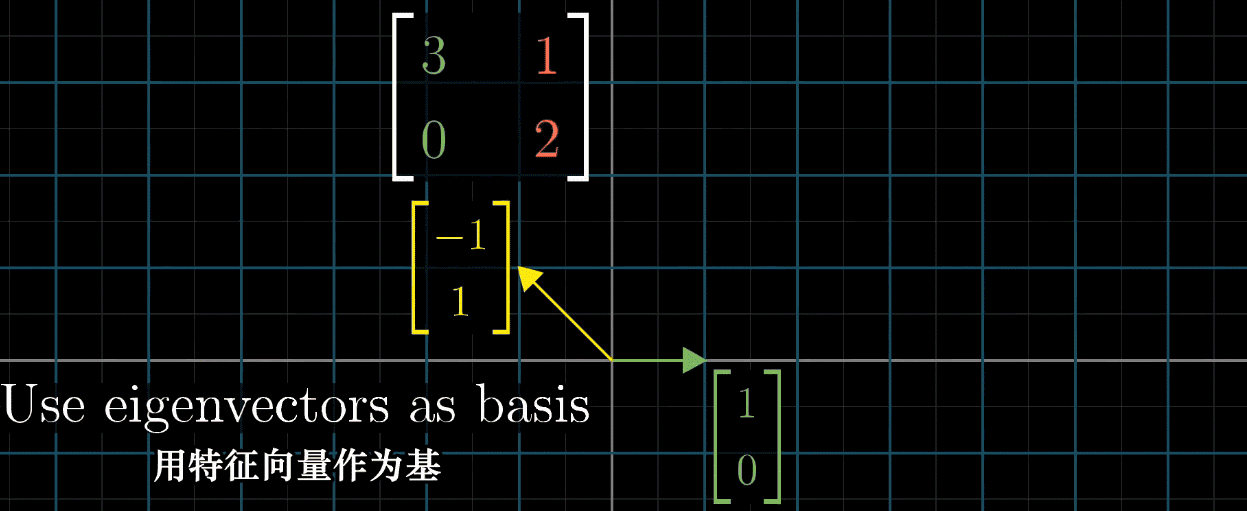# 抽象向量空间

## 函数与向量

$$(f+g)(x) = f(x)+g(x)\\(2f)(x) = 2f(x)$$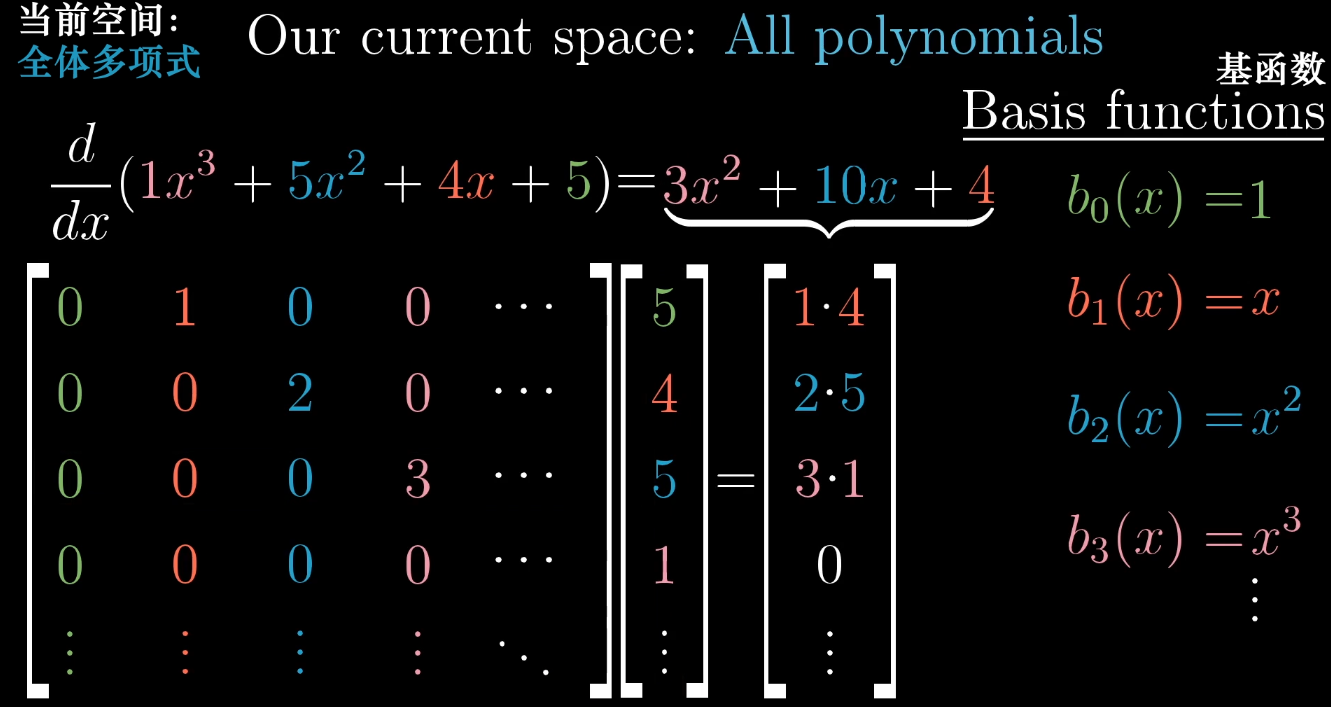## 向量空间